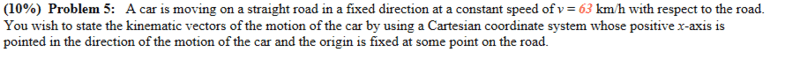# What is the expression for the velocity of the Car in Vector

## Homework Statementv = I + j + k
v = d/t

## The Attempt at a Solution

I thought the answer was as simple as: v = 63i + 0j + 0k, since the car only has motion in one direction...
...but I got it wrong, so clearly I'm missing something here.

#### Attachments

haruspex
Homework Helper
Gold Member
2020 Award
The question is somewhat vague. I see two aspects that are unclear.
What units are to be used in the answer?
Does it want an expression for velocity or one for location?

•Delta2
Delta2
Homework Helper
Gold Member
I think the problem wants the expression for the position vector too, that is ##\vec{r}=r_i\cdot i +0j+0k##. What is ##r_i## here?

Delta2
Homework Helper
Gold Member
it says using the speed v and the unit vectors i,j,k

maybe try ##v\cdot \vec{i} +0\cdot\vec{j}+0\cdot\vec{k}##

•Lapse
Thanks. I knew it was something silly.

•Delta2